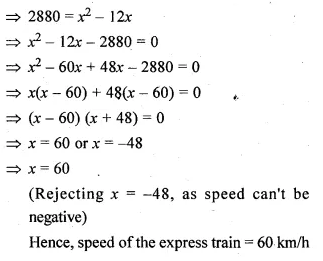Guru

# The speed of an express train is x km/hr and the speed of an ordinary train is 12 km/hr less than that of the express train. If the ordinary train takes one hour longer than the express train to cover a distance of 240 km, find the speed of the express train.

• 0

This question has been taken from Book:- ML aggarwal, Avichal publication, class10th, quadratic equation in one variable, chapter 5, exercise 5.5
This is an important ques

The speed of an express train is x km/hr and the speed of an ordinary train is 12 km/hr less than that of the express train.

If the ordinary train takes one hour longer than the express train to cover a distance of 240 km, find the speed of the express train.
Question no.26 , ML Aggarwal, chapter 5, exercise 5.5, quadratic equation in one variable, ICSE board,

Share